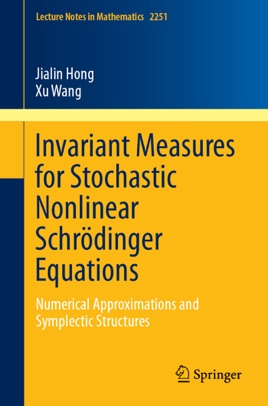• 37,99 €

## Description de l’éditeur

This book provides some recent advance in the study of stochastic nonlinear Schrödinger equations and their numerical approximations, including the well-posedness, ergodicity, symplecticity and multi-symplecticity. It gives an accessible overview of the existence and uniqueness of invariant measures for stochastic differential equations, introduces geometric structures including symplecticity and (conformal) multi-symplecticity for nonlinear Schrödinger equations and their numerical approximations, and studies the properties and convergence errors of numerical methods for stochastic nonlinear Schrödinger equations.
This book will appeal to researchers who are interested in numerical analysis, stochastic analysis, ergodic theory, partial differential equation theory, etc.